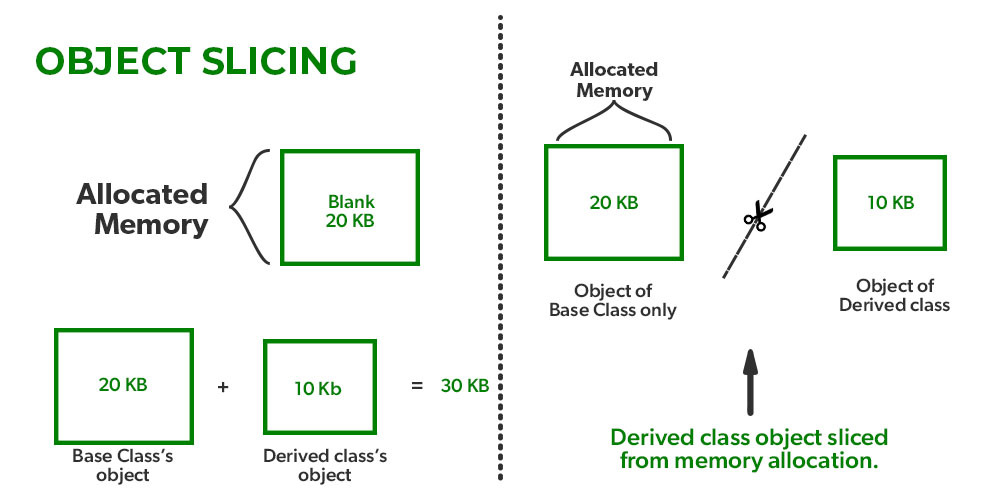GeeksforGeeks App
Open AppBrowser
Continue

# Object Slicing in C++

When a derived class object is assigned to a base class object in C++, the derived class object’s extra attributes are sliced off (not considered) to generate the base class object; and this whole process is termed object slicing. In simple words, when extra components of a derived class are sliced or not used and the priority is given to the base class’s object this is termed object slicing.

In C++, a derived class object can be assigned to a base class object, but the other way is not possible. To tackle this slicing problem we can use a dynamic pointer.

Moreover, Object slicing happens when a derived class object is assigned to a base class object, and additional attributes of a derived class object are sliced off to form the base class object.

## C++

 `// C++ program to demonstrate what is object slicing``class` `Base {``    ``int` `x, y;``};` `class` `Derived : ``public` `Base {``    ``int` `z, w;``};` `int` `main()``{``    ``Derived d;` `    ``// Object Slicing,``    ``// z and w of d are sliced off``    ``Base b = d;``}`Object Slicing

## C++

 `// C++ program to demonstrate the mechanism or working of``// of the object slicing technique``#include ``using` `namespace` `std;` `// Base class``class` `Base {``protected``:``    ``int` `i;` `public``:``    ``Base(``int` `a) { i = a; }``    ``virtual` `void``    ``display() ``// virtual function which is declared in base``              ``// class and re-declared in derived class``    ``{``        ``cout << ``"I am Base class object, i = "` `<< i << endl;``    ``}``};` `// Derived class``class` `Derived : ``public` `Base {``    ``int` `j;` `public``:``    ``Derived(``int` `a, ``int` `b)``        ``: Base(a)``    ``{` `        ``// assigning the value to the data members of``        ``// derived class``        ``j = b;``    ``}``    ``virtual` `void` `display()``    ``{``        ``cout << ``"I am Derived class object, i = "` `<< i``             ``<< ``", j = "` `<< j << endl;``    ``}``};` `// Global method, Base class``// object is passed by value``void` `somefunc(Base obj) { obj.display(); }` `int` `main()``{``    ``Base b(33);``    ``Derived d(45, 54);``    ``somefunc(b);` `    ``// Object Slicing, the member j of d is``    ``// sliced off``    ``somefunc(d);``    ``return` `0;``}`

Output:

```I am Base class object, i = 33
I am Base class object, i = 45```

We can avoid the above-unexpected behavior with the use of pointers or references. Object slicing doesn’t occur when pointers or references to objects are passed as function arguments since a pointer or reference of any type takes the same amount of memory. For example, if we change the global method myfunc() in the above program to the following, object slicing doesn’t happen.

## C++

 `// REFERENCE TO ABOVE` `// rest of code is similar to above``void` `somefunc (Base &obj)``{``    ``obj.display();``}          ``// rest of code is similar to above`

Output:

```I am Base class object, i = 33
I am Derived class object, i = 45, j = 54```

We get the same output if we use pointers.

One of the application of object slicing is seen when an object of derived class is passed to a function which takes object of base class as an argument. This has been demonstrated below.

Example:

## C++

 `// rest of code is similar to above``void` `somefunc (Base *objp)``{``    ``objp->display();``}` `int` `main()``{``    ``Base *bp = ``new` `Base(33) ;``    ``Derived *dp = ``new` `Derived(45, 54);``    ``somefunc(bp);``    ``somefunc(dp);  ``// No Object Slicing``    ``return` `0;``}`

Output:

```I am Base class object, i = 33
I am Derived class object, i = 45, j = 54```

Object slicing can be prevented by making the base class function pure virtual thereby disallowing object creation. It is not possible to create the object of a class that contains a pure virtual method.